Main Content

# Visualizing Microarray Data

This example shows various ways to explore and visualize raw microarray data. The example uses microarray data from a study of gene expression in mouse brains .

### Exploring the Microarray Data Set

Brown, V.M et.al.  used microarrays to explore the gene expression patterns in the brain of a mouse in which a pharmacological model of Parkinson's disease (PD) was induced using methamphetamine. The raw data for this experiment is available from the Gene Expression Omnibus website using the accession number GSE30 .

The file `mouse_h3pd.gpr` contains the data for one of the microarrays used in the study, specifically from a sample collected from voxel H3 of the brain in a Parkinson's Disease (PD) model mouse. The file uses the GenePix® GPR file format. The voxel sample was labeled with Cy3 (green) and the control (RNA from a total, not voxelated, normal mouse brain) was labeled with Cy5.

GPR formatted files provide a large amount of information about the array including the mean, median and standard deviation of the foreground and background intensities of each spot at the 635nm wavelength (the red, Cy5 channel) and the 532nm wavelength (the green, Cy3 channel).

The command `gprread` reads the data from the file into a structure.

```pd = gprread('mouse_h3pd.gpr') ```
```pd = struct with fields: Header: [1x1 struct] Data: [9504x38 double] Blocks: [9504x1 double] Columns: [9504x1 double] Rows: [9504x1 double] Names: {9504x1 cell} IDs: {9504x1 cell} ColumnNames: {38x1 cell} Indices: [132x72 double] Shape: [1x1 struct] ```

You can access the fields of a structure using `dot` notation. For example, access the first ten column names.

```pd.ColumnNames(1:10) ```
```ans = 10x1 cell array {'X' } {'Y' } {'Dia.' } {'F635 Median' } {'F635 Mean' } {'F635 SD' } {'B635 Median' } {'B635 Mean' } {'B635 SD' } {'% > B635+1SD'} ```

You can also access the first ten gene names.

```pd.Names(1:10) ```
```ans = 10x1 cell array {'AA467053'} {'AA388323'} {'AA387625'} {'AA474342'} {'Myo1b' } {'AA473123'} {'AA387579'} {'AA387314'} {'AA467571'} {0x0 char } ```

### Spatial Images of Microarray Data

The `maimage` command can take the microarray data structure and create a pseudocolor image of the data arranged in the same order as the spots on the array, i.e., a spatial plot of the microarray. The "F635 Median" field shows the median pixel values for the foreground of the red (Cy5) channel.

```figure maimage(pd,'F635 Median','title',{'Parkinson''s Model','Foreground Median Pixels','Red Channel'}) ```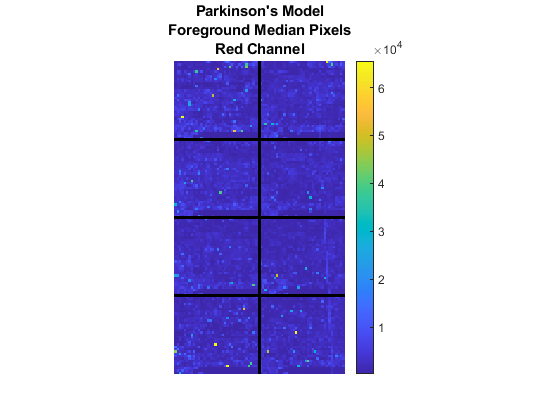The "F532 Median" field corresponds to the foreground of the green (Cy3) channel.

```figure maimage(pd,'F532 Median','title',{'Parkinson''s Model','Foreground Median Pixels','Green Channel'}) ```The "B635 Median" field shows the median values for the background of the red channel. Notice the very high background levels down the right side of the array.

```figure maimage(pd,'B635 Median','title',{'Parkinson''s Model','Background Median Pixels','Red Channel'}) ```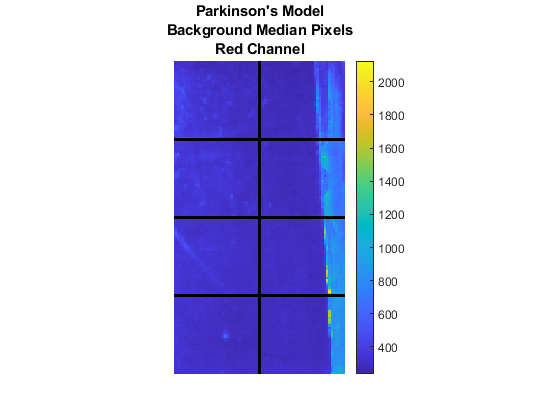The "B532 Median" shows the median values for the background of the green channel.

```figure maimage(pd,'B532 Median','title',{'Parkinson''s Model','Background Median Pixels','Green Channel'}) ```You can now consider the data obtained for the same brain voxel in an untreated control mouse. In this case, the voxel sample was labeled with Cy3, and the control (RNA from a total, not voxelated brain) was labeled with Cy5.

```wt = gprread('mouse_h3wt.gpr') ```
```wt = struct with fields: Header: [1x1 struct] Data: [9504x38 double] Blocks: [9504x1 double] Columns: [9504x1 double] Rows: [9504x1 double] Names: {9504x1 cell} IDs: {9504x1 cell} ColumnNames: {38x1 cell} Indices: [132x72 double] Shape: [1x1 struct] ```

Use `maimage` to show pseudocolor images of the foreground and background corresponding to the untreated mouse. The `subplot` command can be used to combine the plots.

```figure subplot(2,2,1); maimage(wt,'F635 Median','title',{'Foreground','(Red)'}) subplot(2,2,2); maimage(wt,'F532 Median','title',{'Foreground','(Green)'}) subplot(2,2,3); maimage(wt,'B635 Median','title',{'Background','(Red)'}) subplot(2,2,4); maimage(wt,'B532 Median','title',{'Background','(Green)'}) annotation('textbox','String','Wild Type Median Pixel Values', ... 'Position', [0.3 0.05 0.9 0.01],'EdgeColor','none','FontSize',12); ```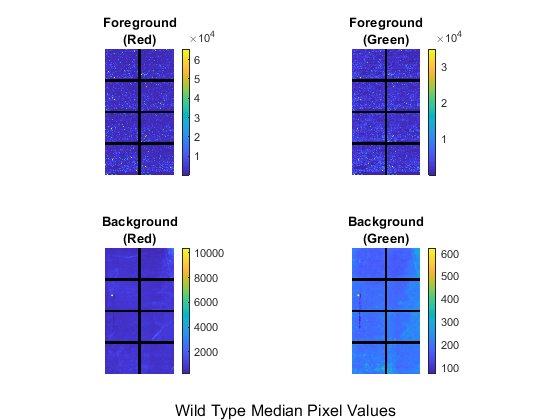If you look at the scale for the background images, you will notice that the background levels are much higher than those for the PD mouse and there appears to be something non random affecting the background of the Cy3 channel of this slide. Changing the colormap can sometimes provide more insight into what is going on in pseudocolor plots. For more control over the color, try the `colormapeditor` function. You can also right-click on the colorbar to bring up various options for modifying the colormap of the plot including interactive colormap shifting.

```colormap hot ```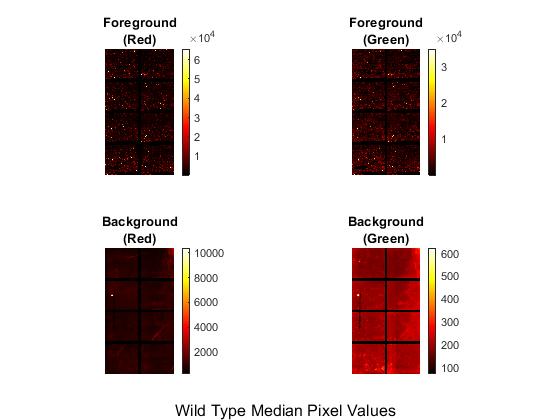The `maimage` command is a simple way to quickly create pseudocolor images of microarray data. However, sometimes it is convenient to create customizable plots using the `imagesc` command, as shown below.

Use `magetfield` to extract data for the B532 median field and the Indices field to index into the Data. You can bound the intensities of the background plot to give more contrast in the image.

```b532Data = magetfield(wt,'B532 Median'); maskedData = b532Data; maskedData(b532Data<500) = 500; maskedData(b532Data>2000) = 2000; figure subplot(1,2,1); imagesc(b532Data(wt.Indices)) axis image colorbar title('B532, WT') subplot(1,2,2); imagesc(maskedData(wt.Indices)) axis image colorbar title('Enhanced B532, WT') ```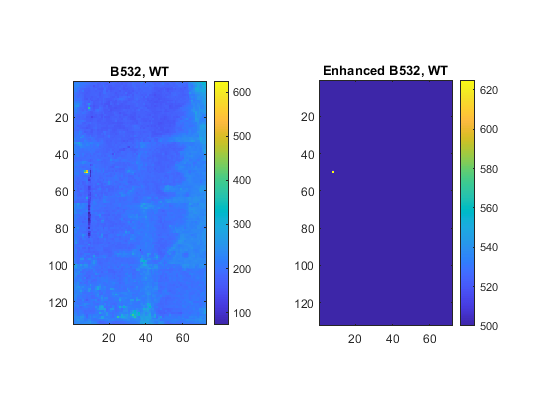### Statistics of the Microarrays

The `maboxplot` function can be used to look at the distribution of data in each of the blocks.

```figure subplot(2,1,1) maboxplot(pd,'F532 Median','title','Parkinson''s Disease Model Mouse') subplot(2,1,2) maboxplot(pd,'B532 Median','title','Parkinson''s Disease Model Mouse') figure subplot(2,1,1) maboxplot(wt,'F532 Median','title','Untreated Mouse') subplot(2,1,2) maboxplot(wt,'B532 Median','title','Untreated Mouse') ```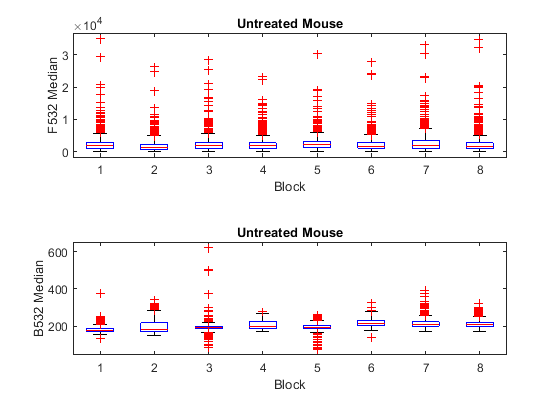From the box plots you can clearly see the spatial effects in the background intensities. Blocks number 1,3,5 and 7 are on the left side of the arrays, and blocks number 2,4,6 and 8 are on the right side.

There are two columns in the microarray data structure labeled "F635 Median - B635" and "F532 Median - B532". These columns are the differences between the median foreground and the median background for the 635 nm channel and 532 nm channel respectively. These give a measure of the actual expression levels. The spatial effect is less noticeable in these plots.

```figure subplot(2,1,1) maboxplot(pd,'F635 Median - B635','title','Parkinson''s Disease Model Mouse ') subplot(2,1,2) maboxplot(pd,'F532 Median - B532','title','Parkinson''s Disease Model Mouse') figure subplot(2,1,1) maboxplot(wt,'F635 Median - B635','title','Untreated Mouse') subplot(2,1,2) maboxplot(wt,'F532 Median - B532','title','Untreated Mouse') ```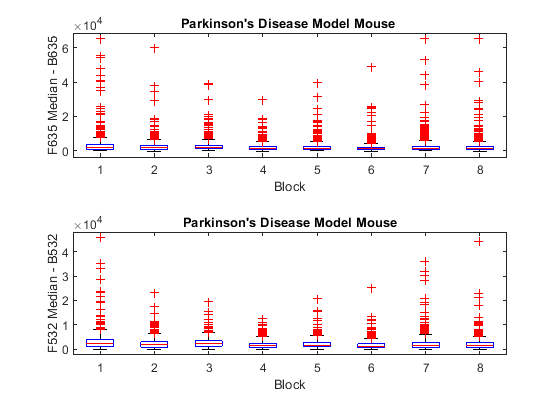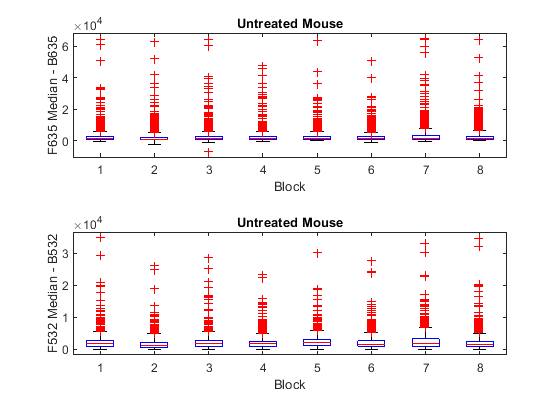### Scatter Plots of Microarray Data

Rather than work with the data in the larger structure, it is often easier to extract the data into separate variables.

```cy5Data = magetfield(pd,'F635 Median - B635'); cy3Data = magetfield(pd,'F532 Median - B532'); ```

A simple way to compare the two channels is with a `loglog` plot. The function `maloglog` is used to do this. Points that are above the diagonal in this plot correspond to genes that have higher expression levels in the H3 voxel than in the brain as a whole.

```figure maloglog(cy5Data,cy3Data) title('Loglog Scatter Plot of PD Model'); xlabel('F635 Median - B635 (Control)'); ylabel('F532 Median - B532 (Voxel H3)'); ```
```Warning: Zero values are ignored. Warning: Negative values are ignored. ```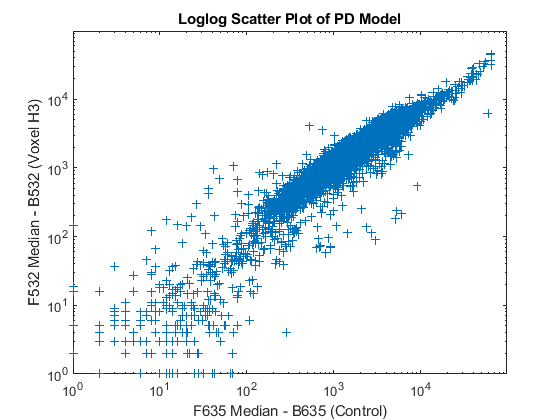Notice how the `loglog` function gives some warnings about negative and zero elements. This is because some of the values in the 'F635 Median - B635' and 'F532 Median - B532' columns are zero or less than zero. Spots where this happened might be bad spots or spots that failed to hybridize. Similarly, spots with positive, but very small, differences between foreground and background are also considered bad spots. These warnings can be disabled using the `warning` command.

```warnState = warning; % Save the current warning state warning('off','bioinfo:maloglog:ZeroValues'); warning('off','bioinfo:maloglog:NegativeValues'); figure maloglog(cy5Data,cy3Data) title('Loglog Scatter Plot of PD Model'); xlabel('F635 Median - B635 (Control)'); ylabel('F532 Median - B532 (Voxel H3)'); warning(warnState); % Reset the warning state ```An alternative to simply ignoring or disabling the warnings is to remove the bad spots from the data set. This can be done by finding points where either the red or green channel have values less than or equal to a threshold value, for example 10.

```threshold = 10; badPoints = (cy5Data <= threshold) | (cy3Data <= threshold); ```

You can then remove these points and redraw the loglog plot.

```cy5Data(badPoints) = []; cy3Data(badPoints) = []; figure maloglog(cy5Data,cy3Data) title('Refined Loglog Scatter Plot of PD Model'); xlabel('F635 Median - B635 (Control)'); ylabel('F532 Median - B532 (Voxel H3)'); ```The distribution plot can be annotated by labeling the various points with the corresponding genes.

```figure maloglog(cy5Data,cy3Data,'labels',pd.Names(~badPoints),'factorlines',2) title('Loglog Scatter Plot of PD Model'); xlabel('F635 Median - B635 (Control)'); ylabel('F532 Median - B532 (Voxel H3)'); ```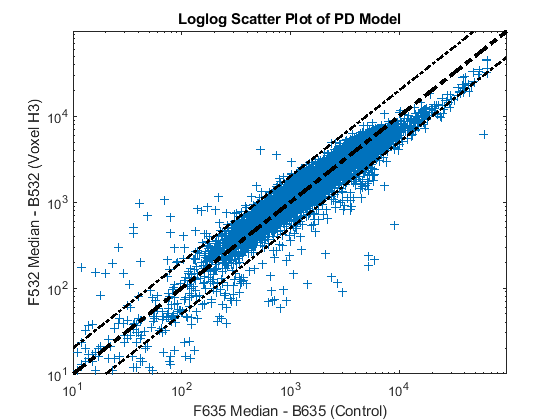Try using the mouse to click on some of the outlier points. You will see the gene name associated with the point. Most of the outliers are below the y = x line. In fact most of the points are below this line. Ideally the points should be evenly distributed on either side of this line. In order for this to happen, the points need to be normalized. You can use the `manorm` function to perform global mean normalization.

```normcy5 = manorm(cy5Data); normcy3 = manorm(cy3Data); ```

If you plot the normalized data you will see that the points are more evenly distributed about the y = x line.

```figure maloglog(normcy5,normcy3,'labels',pd.Names(~badPoints),'factorlines',2) title('Normalized Loglog Scatter Plot of PD Model'); xlabel('F635 Median - B635 (Control)'); ylabel('F532 Median - B532 (Voxel H3)'); ```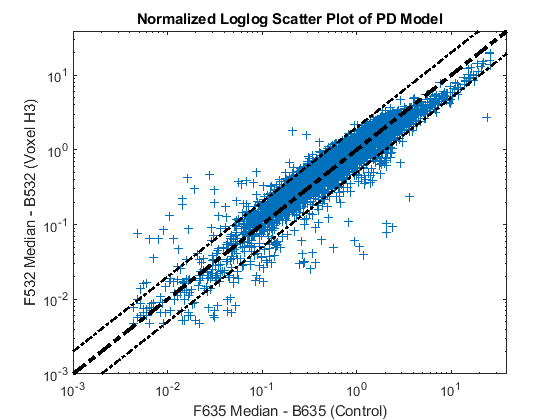You will recall that the background of the chips was not uniform. You can use print-tip (block) normalization to normalize each block separately. The function `manorm` will perform block normalization automatically if block information is available in the microarray data structure.

```bn_cy5Data = manorm(pd,'F635 Median - B635'); bn_cy3Data = manorm(pd,'F532 Median - B532'); ```

Instead of removing negative or points below the threshold, you can set them to NaN. This does not change the size or shape of the data, but NaN points will not be displayed on plots.

```bn_cy5Data(bn_cy5Data <= 0) = NaN; bn_cy3Data(bn_cy3Data <= 0) = NaN; figure maloglog(bn_cy5Data,bn_cy3Data,'labels',pd.Names,'factorlines',2) title('Refined, Normalized Loglog Scatter Plot of PD Model'); xlabel('F635 Median - B635 (Control)'); ylabel('F532 Median - B532 (Voxel H3)'); ```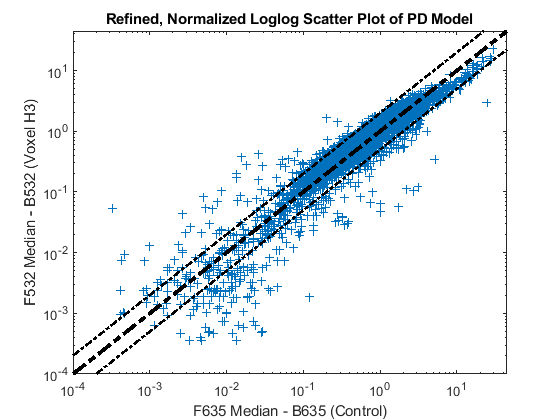The function `mairplot` is used to create an Intensity vs. Ratio plot for the normalized data. If the name-value pair 'PlotOnly' is set to false, you can explore the data interactively, such as select points to see the names of the associated genes, normalize the data, highlight gene names in the up-regulated or down-regulated lists, or change the values of the factor lines.

```mairplot(normcy5,normcy3,'labels',pd.Names(~badPoints),'PlotOnly',true,... 'title','Intensity vs. Ratio of PD Model'); ```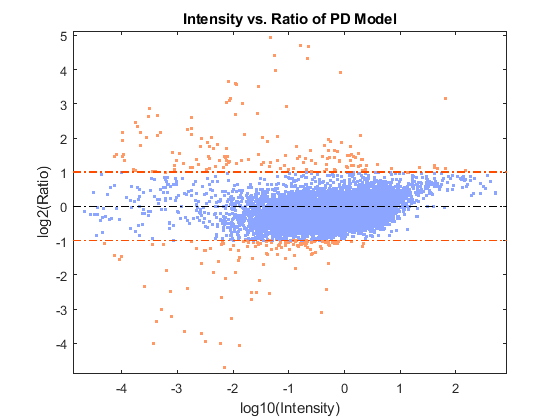You can use the `Normalize` option to `mairplot` to perform Lowess normalization on the data.

```mairplot(normcy5,normcy3,'labels',pd.Names(~badPoints),'PlotOnly',true,... 'Normalize',true,'title', 'Intensity vs. Ratio of PD Model (Normalized)'); ````GenePix is a registered trademark of Axon Instruments, Inc.`

### References

 Brown, V.M., et al., "Multiplex three dimensional brain gene expression mapping in a mouse model of Parkinson's disease", Genome Research, 12(6):868-84, 2002.

## SupportGet trial now